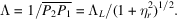International
Tables for
Crystallography
Volume B
Reciprocal space
Edited by U. Shmueli

International Tables for Crystallography (2006). Vol. B, ch. 5.1, pp. 542-543   | 1 | 2 |

Section 5.1.6.3. Boundary conditions at the exit surface

A. Authiera*

aLaboratoire de Minéralogie-Cristallographie, Université P. et M. Curie, 4 Place Jussieu, F-75252 Paris CEDEX 05, France
Correspondence e-mail: authier@lmcp.jussieu.fr

| top | pdf |

5.1.6.3.1. Wavevectors

| top | pdf |

When a wavefield reaches the exit surface, it breaks up into its two constituent waves. Their wavevectors are obtained by applying again the condition of the continuity of their tangential components along the crystal surface. The extremities,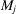and, of these wavevectors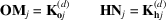lie at the intersections of the spheres of radius k centred at O and H, respectively, with the normal n′ to the crystal exit surface drawn from(j = 1 and 2) (Fig. 5.1.6.3).Figure 5.1.6.3 | top | pdf |Boundary condition for the wavevectors at the exit surface. (a) Reciprocal space. The wavevectors of the emerging waves are determined by the intersections,,andof the normals n′ to the exit surface, drawn from the tie pointsandof the wavefields, with the tangents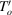and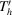to the spheres centred at O and H and of radius k, respectively. (b) Direct space.

If the crystal is wedge-shaped and the normals n and n′ to the entrance and exit surfaces are not parallel, the wavevectors of the waves generated by the two wavefields are not parallel. This effect is due to the refraction properties associated with the dispersion surface.

5.1.6.3.2. Amplitudes – Pendellösung

| top | pdf |

We shall assume from now on that the crystal is plane parallel. Two wavefields arrive at any point of the exit surface. Their constituent waves interfere and generate emerging waves in the refracted and reflected directions (Fig. 5.1.6.4). Their respective amplitudes are given by the boundary conditions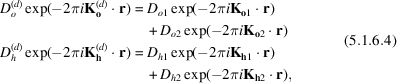where r is the position vector of a point on the exit surface, the origin of phases being taken at the entrance surface.Figure 5.1.6.4 | top | pdf |Decomposition of a wavefield into its two components when it reaches the exit surface.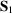and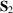are the Poynting vectors of the two wavefields propagating in the crystal belonging to branches 1 and 2 of the dispersion surface, respectively, and interfering at the exit surface.

In a plane-parallel crystal, (5.1.6.4)reduces to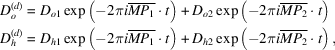where t is the crystal thickness.

In a non-absorbing crystal, the amplitudes squared are of the form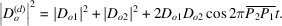This expression shows that the intensities of the refracted and reflected beams are oscillating functions of crystal thickness. The period of the oscillations is called the Pendellösung distance and is